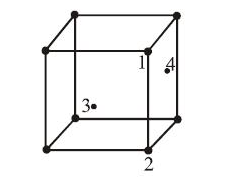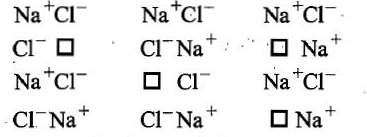In an f.c.c. unit cell. atoms are numbered as shown below.The atoms not touching each other are (Atom numbered 3 is face centre of front face).(A) 3&4
(B) 1&3
(C) 1&2
(D) 2&4

Concept Questions :-

Introduction and Type of Crystal System
High Yielding Test Series + Question Bank - NEET 2020

Difficulty Level:

A solid has a bcc structure. If the distance of closest approach between the two atoms is 1.73Å . The edge length of the cell is:

(a) 200pm

(b) $\sqrt{3}}{\sqrt{2}}$ pm

(c) 142.2 pm

(d) $\sqrt{2}$pm

Concept Questions :-

Density/Formula/packing fraction/ Semiconductors
High Yielding Test Series + Question Bank - NEET 2020

Difficulty Level:

Which is covalent solid?

(a) Fe2O3

(b) Diamond

(c) Graphite

(d) All of these

Concept Questions :-

Introduction and Type of Crystal System
High Yielding Test Series + Question Bank - NEET 2020

Difficulty Level:

What type of crystal defects is indicated in the diagram given below?(a) Frenkel and Schottky defects
(b) Schottky defect
(c) Interstitial defect
(d) Frenkel defect

Concept Questions :-

Imperfections in Solids
High Yielding Test Series + Question Bank - NEET 2020

Difficulty Level:

The anions (A) form hexagonal closest packing and atoms (C) occupy only 2/3 of octahedral voids in
it. then the general formula of the compound is-

(A) CA

(B) A2

(C) C2A3

(D) C3A2

Concept Questions :-

Density/Formula/packing fraction/ Semiconductors
High Yielding Test Series + Question Bank - NEET 2020

Difficulty Level:

The mass of a unit cell of CsCl corresponds to:-

1. 8Cs+ and Cl-

2. 1Cs+ and 6Cl-

3. 1Cs+ and 1Cl-

4. 4Cs+ and Cl-

Concept Questions :-

Introduction and Type of Crystal System
High Yielding Test Series + Question Bank - NEET 2020

Difficulty Level:

Graphite in an example of-

(A) Ionic solid

(B) Covalent solid

(C) Vander waal's crystal

(D) Metallic crystal

Concept Questions :-

Introduction and Type of Crystal System
High Yielding Test Series + Question Bank - NEET 2020

Difficulty Level:

Which arrangement of electrons leads to anti-ferromagnetism?
(a)  ↑↑↑↑
(b)  ↑↓↑↓
(c) Both (a) and (b)
(d) None of these

Concept Questions :-

Magnetic Behaviour of an Element/Compound
High Yielding Test Series + Question Bank - NEET 2020

Difficulty Level:

The intermetallic compound LiAg has a cubic crystalline structure in which each Li atom has 8 nearest neighbour silver atoms and vice-versa. What is the type of unit cell?

1. Body centred cubic

2. Face centred cubic

3. Simple cubic for either Li atoms alone or Ag atoms alone

4. None of the above

Concept Questions :-

Introduction and Type of Crystal System
High Yielding Test Series + Question Bank - NEET 2020

Difficulty Level:

The arrangement ABC ABC.. is referred to as.

1. octahedral close packing

2. hexagonal clase packing

3. tetrahedral close packing

4. cubic close packing

Concept Questions :-